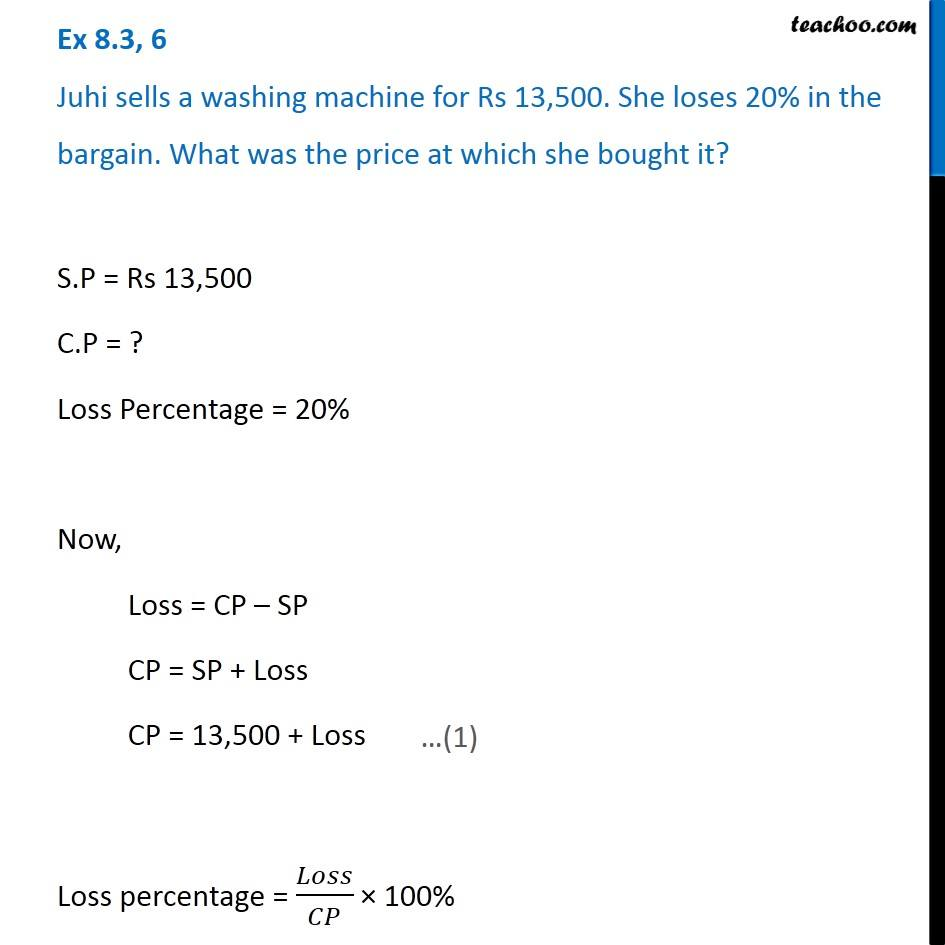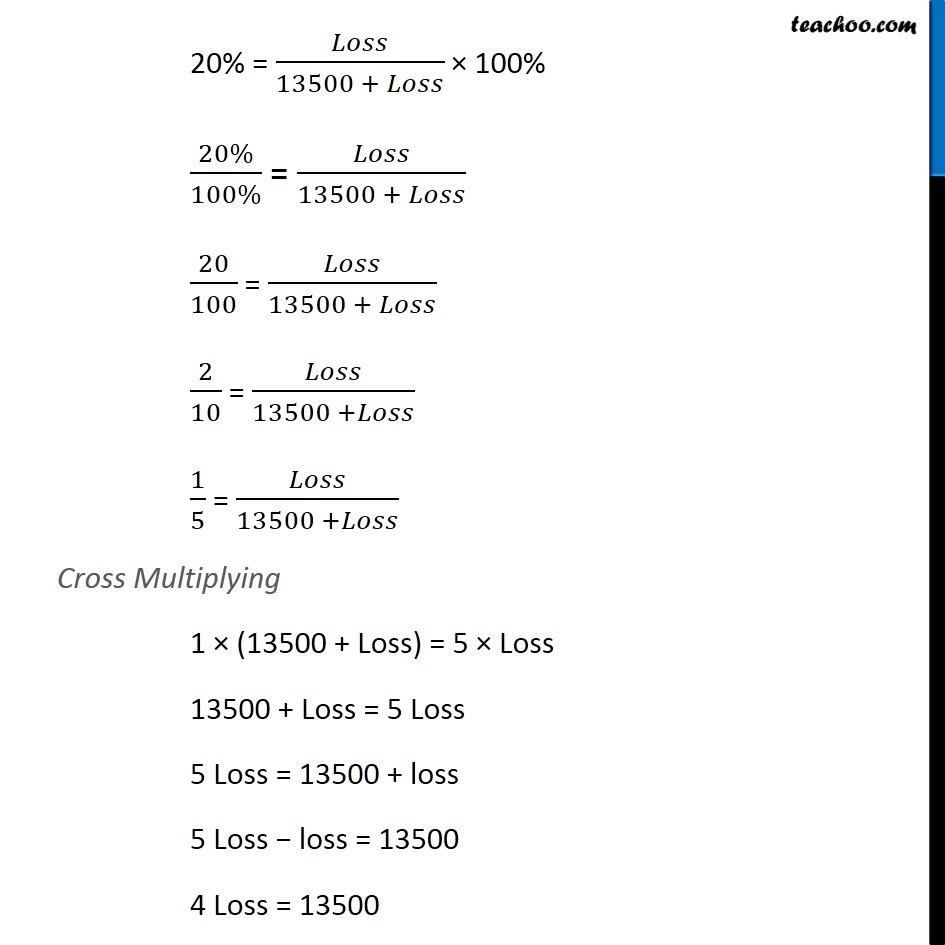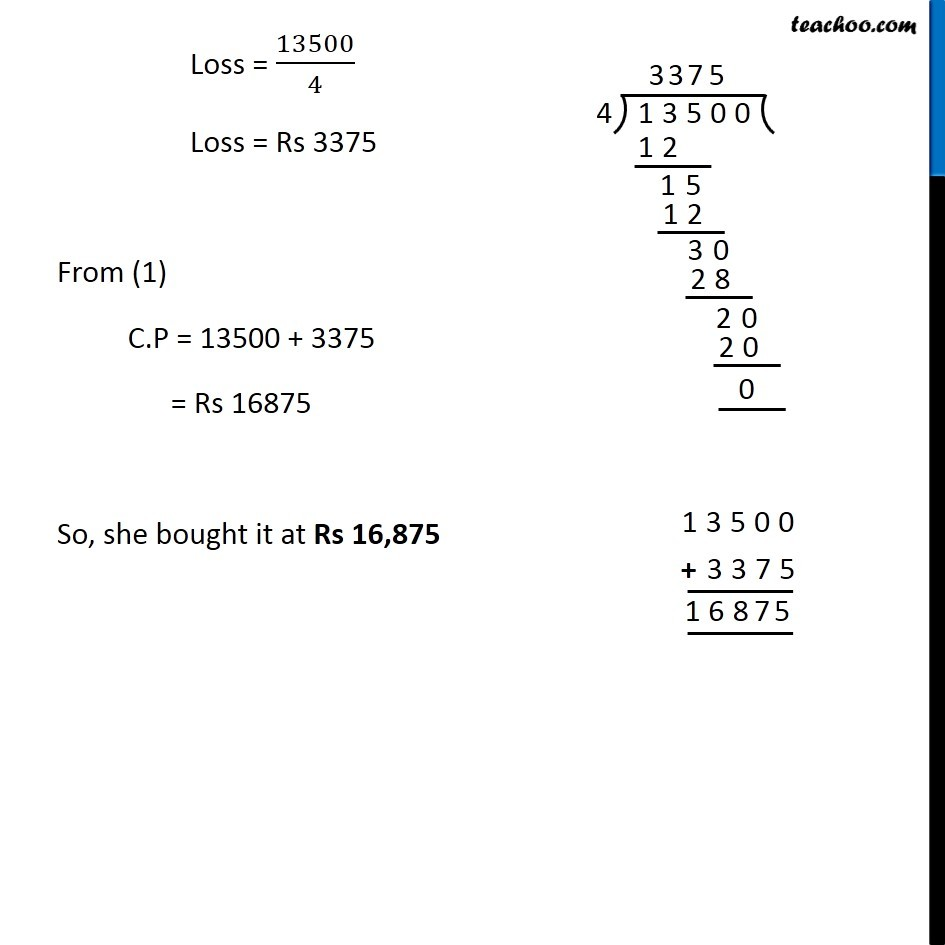Cost price, selling price, profit % , loss%

Chapter 7 Class 7 Comparing Quantities
Concept wiseLearn in your speed, with individual attention - Teachoo Maths 1-on-1 Class

### Transcript

Ex 7.2, 6 Juhi sells a washing machine for Rs 13,500. She loses 20% in the bargain. What was the price at which she bought it? S.P = Rs 13,500 C.P = ? Loss Percentage = 20% Now, Loss = CP – SP CP = SP + Loss CP = 13,500 + Loss …(1) Loss percentage = 𝐿𝑜𝑠𝑠/𝐶𝑃 × 100% 20% = 𝐿𝑜𝑠𝑠/(13500 + 𝐿𝑜𝑠𝑠) × 100% (20%)/(100%) = 𝐿𝑜𝑠𝑠/(13500 + 𝐿𝑜𝑠𝑠) 20/100 = 𝐿𝑜𝑠𝑠/(13500 + 𝐿𝑜𝑠𝑠) 2/10 = 𝐿𝑜𝑠𝑠/(13500 +𝐿𝑜𝑠𝑠) 1/5 = 𝐿𝑜𝑠𝑠/(13500 +𝐿𝑜𝑠𝑠) Cross Multiplying 1 × (13500 + Loss) = 5 × Loss 13500 + Loss = 5 Loss 5 Loss = 13500 + loss 5 Loss − loss = 13500 4 Loss = 13500 Loss = 13500/4 Loss = Rs 3375 From (1) C.P = 13500 + 3375 = Rs 16875 So, she bought it at Rs 16,875